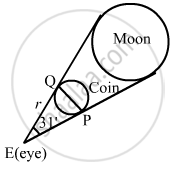Advertisement Remove all ads

# Find the Distance from the Eye at Which a Coin of 2 Cm Diameter Should Be Held So as to Conceal the Full Moon Whose Angular Diameter is 31'. - Mathematics

Find the distance from the eye at which a coin of 2 cm diameter should be held so as to conceal the full moon whose angular diameter is 31'.

Advertisement Remove all ads

#### Solution

Let PQ be the diameter of the coin and E be the eye of the observer.
Also, let the coin be kept at a distance r from the eye of the observer to hide the moon completely.
Now,

$\theta = 31' = \left( \frac{31}{60} \right)^\circ = \left( \frac{31}{60} \times \frac{\pi}{180} \right) \text{ radians }$

$\theta = \frac{\text{Arc}}{\text{Radius}}$

$\Rightarrow \frac{31}{60} \times \frac{\pi}{180} = \frac{2}{\text{Radius}}$

$\Rightarrow\text{ Radius }= \frac{180 \times 60 \times 2 \times 7}{31 \times 22}$

$= 221 . 7\text{ cm or }2 . 217 m$Is there an error in this question or solution?
Advertisement Remove all ads

#### APPEARS IN

RD Sharma Class 11 Mathematics Textbook
Chapter 4 Measurement of Angles
Exercise 4.1 | Q 17 | Page 16
Advertisement Remove all ads

#### Video TutorialsVIEW ALL 

Advertisement Remove all ads
Share
Notifications

View all notifications

Forgot password?